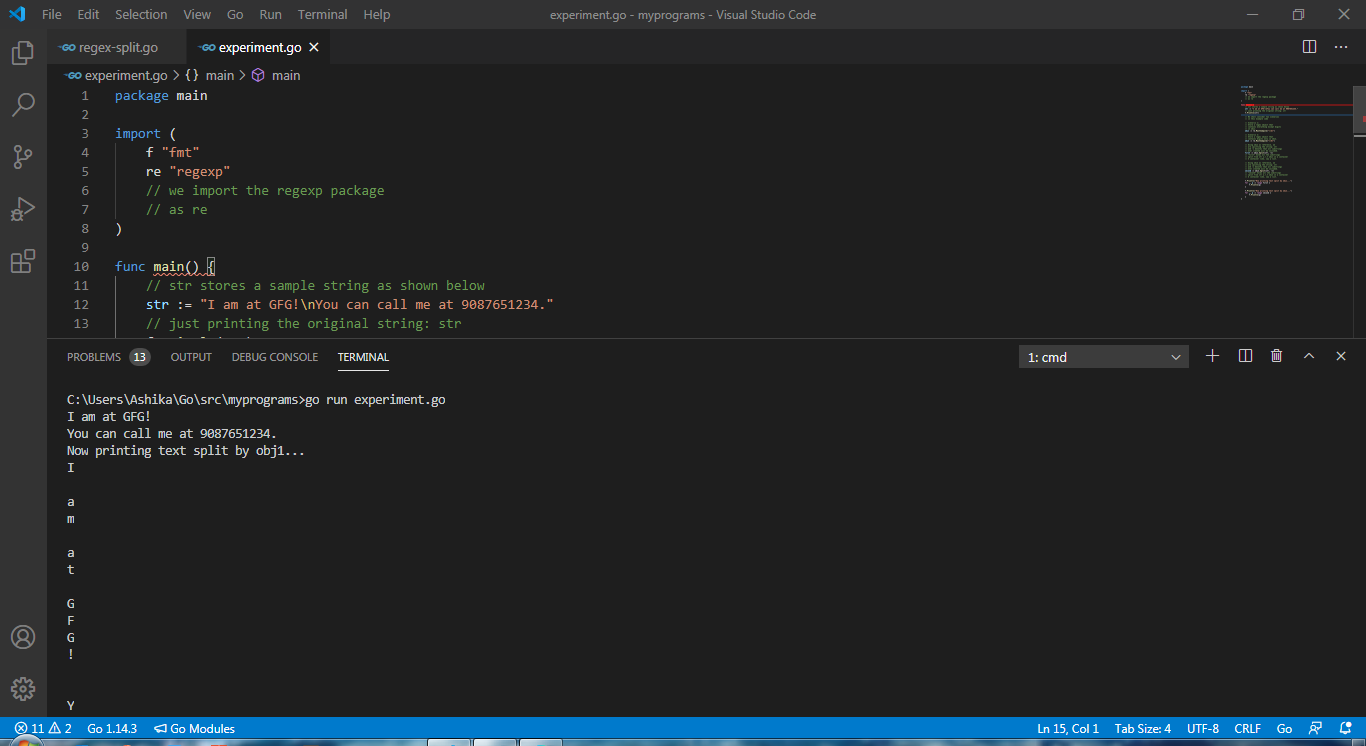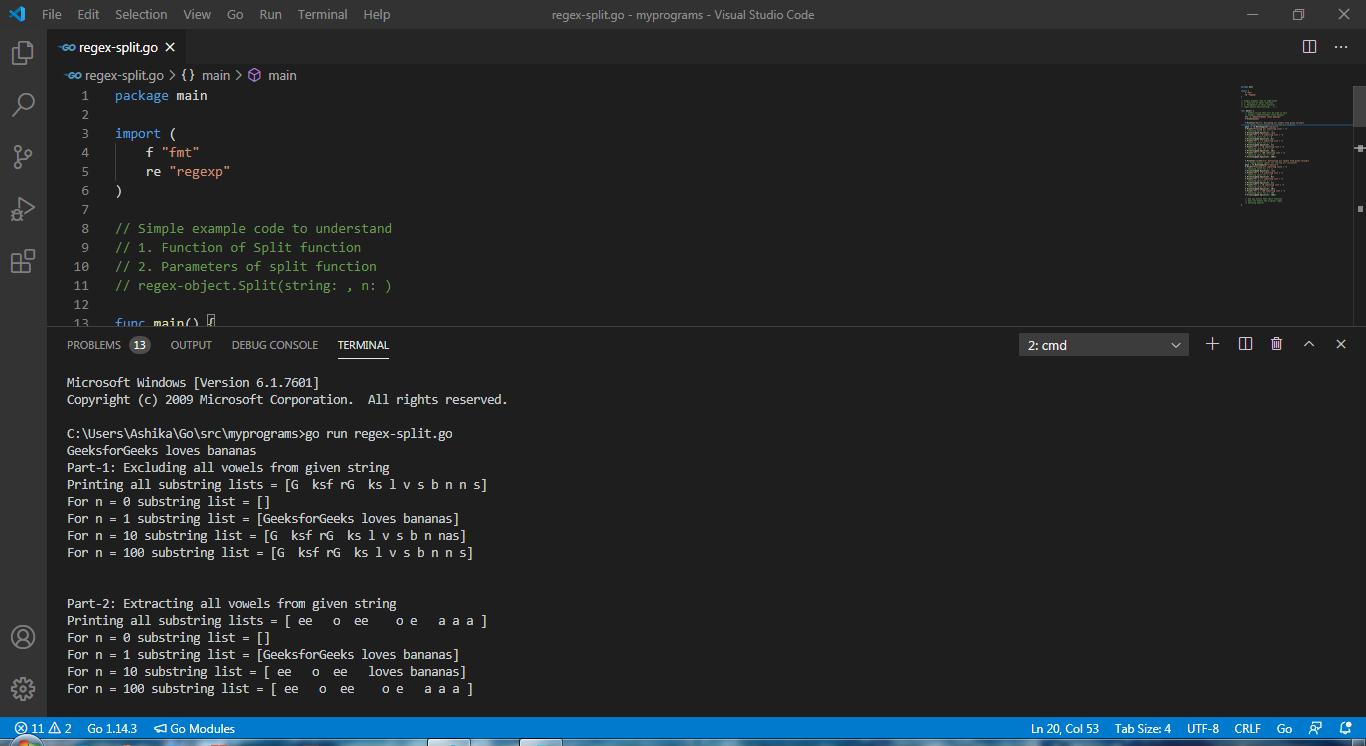Open In App

# How to Split Text Using Regex in Golang?

What is regex? Is Regex the famous anime character? Oops, if you think so, you’re likely to be disappointed. The Go programming language uses the term regexp to denote Regular expressions. Regular expressions are very important in the field of string processing. The “regexp” package in Go holds all necessary pre-built functions that implement regular expression search and also guarantee a linear-time search in the size of the provided inputs. To know more about what regex is, read What is Regexp in Golang?

### How to split input text using regexp (or regex)?

Regexp package contains the Split function which helps in splitting the input text string. Before we jump into the depths of regexp split function, let us brief you over some basic regular expressions that are worth remembering while using the split function:

Syntax:

```func Split(s: string, n: int) []string
```

This function accepts a string and an integer and returns a slice of all substrings. The input string ‘s‘ is the string that will be further, split into substrings as per the given regular expression by Split function. ‘n‘ denotes the value that decides the number of substrings to be returned.

• If n > 0: It means that a maximum of n substrings undergo the regex operation and will be returned.
• If n = 1 then no regex the operation will be performed and hence, the original string will be returned.
• If n = 0: It means that no substrings will be returned, a nill shall be returned.
• If n < 0: It means that all the substrings  that was created will be returned by the function.

Example 1:

## Go

 `package` `main`` ` `import` `(``    ``f ``"fmt"``    ``re ``"regexp"``    ``// we import the regexp package``    ``// as re``)`` ` `// SPLIT function hacks``// In layman terms, whenever the``// string (given to split as argument)``// occurs, the string is split into``// a substring.`` ` `func` `main() {`` ` `    ``// str stores a sample string as shown below``    ``str := ``"I am at GFG!\nYou can call me at 9087651234."``     ` `    ``// just printing the original string: str``    ``f.Println(str)`` ` `    ``// We shall consider two scenarios``    ``// in this example code`` ` `    ``// Scenario 1:``    ``// Store a regex object that``    ``// contains digits only``    ``// in obj1.``    ``obj1 := re.MustCompile(``"\\d*"``)`` ` `    ``// Scenario 2:``    ``// Store a regex object that``    ``// contains everything except digits``    ``// in obj2.``    ``obj2 := re.MustCompile(``"\\D*"``)`` ` `    ``// Using obj1 as reference, we``    ``// are splitting the string: str``    ``// and -1 denotes that all substrings``    ``// ever created will be included.``    ``first := obj1.Split(str, -``1``)``     ` `    ``// "first" holds all the substrings``    ``// split from str w.r.t obj1 in a container``    ``// A container like, say a list.`` ` `    ``// Using obj2 as reference, we``    ``// are splitting the string: str``    ``// and -1 denotes that all substrings``    ``// ever created will be included.``    ``second := obj2.Split(str, -``1``)``    ``// "second" holds all the substrings``    ``// split from str w.r.t obj2 in a container``    ``// A container like, say a list.`` ` `    ``f.Println(``"Now printing text split by obj1..."``)``    ``for` `_, p := ``range` `first {``        ``f.Println(p)``    ``}`` ` `    ``f.Println(``"Now printing text split by obj2..."``)``    ``for` `_, q := ``range` `second {``        ``f.Println(q)``    ``}``}`

Command to Execute:

```> go run (your_file_name).go
```

Output:

```I am at GFG!
You can call me at 9087651234.
Now printing text split by obj1...
I

a
m

a
t

G
F
G
!

Y
o
u

c
a
n

c
a
l
l

m
e

a
t

.
Now printing text split by obj2...

9
0
8
7
6
5
1
2
3
4
```

Visual I/O demo on Visual Studio Code:Code output on-screen upon running the above-written code.

Example 2:

## Go

 `package` `main`` ` `import` `(``    ``f ``"fmt"``    ``re ``"regexp"``)`` ` `// Simple example code to understand``// 1. Function of Split function``// 2. Parameters of split function``// regex-object.Split(string: , n: )`` ` `func` `main() {`` ` `    ``// Sample string that will be used in this``    ``// example "GeeksforGeeks loves bananas"``    ``str := ``"GeeksforGeeks loves bananas"``    ``f.Println(str)`` ` `    ``f.Println(``"Part-1: Excluding all vowels from given string"``)``     ` `    ``// a regexp object (geek) for storing all vowels``    ``geek := re.MustCompile(``"[aeiou]"``)``    ``f.Print(``"Printing all substring lists = "``)``     ` `    ``// Checking split for n = -1``    ``f.Println(geek.Split(str, -``1``))``    ``f.Print(``"For n = 0 substring list = "``)``     ` `    ``// Checking split for n = 0``    ``f.Println(geek.Split(str, ``0``))``    ``f.Print(``"For n = 1 substring list = "``)``     ` `    ``// Checking split for n = 1``    ``f.Println(geek.Split(str, ``1``))``    ``f.Print(``"For n = 10 substring list = "``)``     ` `    ``// Checking split for n = 10``    ``f.Println(geek.Split(str, ``10``))``    ``f.Print(``"For n = 100 substring list = "``)``     ` `    ``// Checking split for n = 100``    ``f.Println(geek.Split(str, ``100``))`` ` `    ``f.Println(``"\n\nPart-2: Extracting all vowels from given string"``)``     ` `    ``// a regexp object (geek) for storing all consonants``    ``geek = re.MustCompile(``"[^aeiou]"``)``     ` `    ``f.Print(``"Printing all substring lists = "``)``     ` `    ``// Checking split for n = -1``    ``f.Println(geek.Split(str, -``1``))``    ``f.Print(``"For n = 0 substring list = "``)``     ` `    ``// Checking split for n = 0``    ``f.Println(geek.Split(str, ``0``))``    ``f.Print(``"For n = 1 substring list = "``)``     ` `    ``// Checking split for n = 1``    ``f.Println(geek.Split(str, ``1``))``    ``f.Print(``"For n = 10 substring list = "``)``     ` `    ``// Checking split for n = 10``    ``f.Println(geek.Split(str, ``10``))``    ``f.Print(``"For n = 100 substring list = "``)``     ` `    ``// Checking split for n = 100``    ``f.Println(geek.Split(str, ``100``))`` ` `    ``// Did you notice that split function``    ``// does not modify the original regex``    ``// matching object?``}`

Command to Execute:

```> go run (your_file_name).go
```

Output:

```GeeksforGeeks loves bananas
Part-1: Excluding all vowels from given string
Printing all substring lists = [G  ksf rG  ks l v s b n n s]
For n = 0 substring list = []
For n = 1 substring list = [GeeksforGeeks loves bananas]
For n = 10 substring list = [G  ksf rG  ks l v s b n nas]
For n = 100 substring list = [G  ksf rG  ks l v s b n n s]

Part-2: Extracting all vowels from given string
Printing all substring lists = [ ee   o  ee    o e   a a a ]
For n = 0 substring list = []
For n = 1 substring list = [GeeksforGeeks loves bananas]
For n = 10 substring list = [ ee   o  ee   loves bananas]
For n = 100 substring list = [ ee   o  ee    o e   a a a ]
```

Visual I/O demo on Visual Studio Code:Code output on-screen upon running the above-written code.